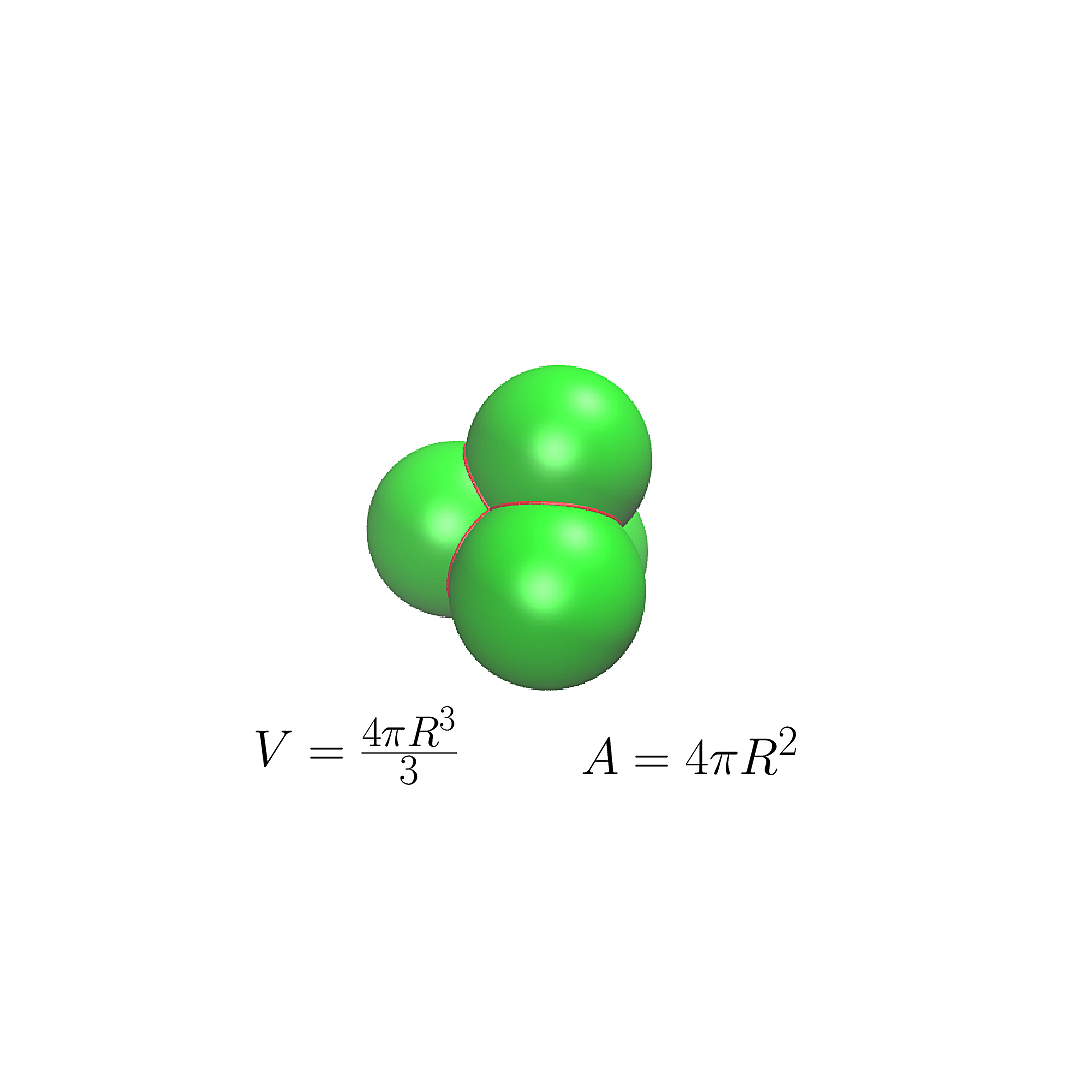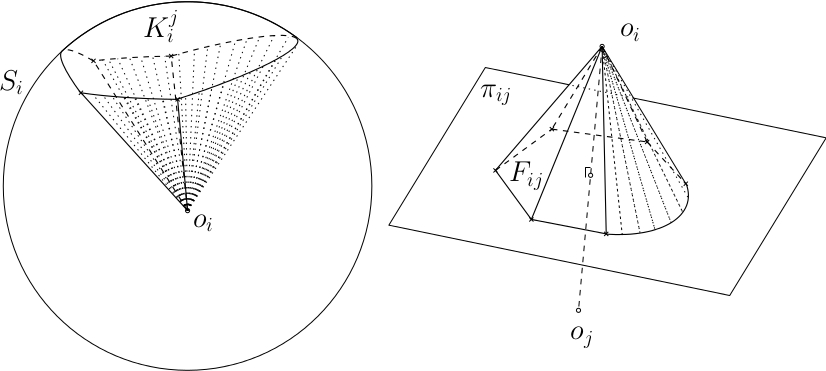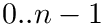Structural Bioinformatics Library Template C++ / Python API for developping structural bioinformatics applications.
User Manual# Union_of_balls_surface_volume_3

Authors: F. Cazals and T. Dreyfus and S. Loriot

# Introduction

This package provides a generic geometric data structure and algorithm for computing the surface area and the volume of the union of a family of 3D balls. Compared to other algorithms, it warranties:

• time efficiency (...)
• bounded errors.

Calculations are handled in a "as most as possible" exact (due to trigonometric functions, the volume and surface area cannot be exact), or using interval arithmetics.

# Algorithms

## Surfaces and Volumes

Having commented above on the decomposition of a union of balls into restrictions, it is sufficient to comment on the geometry of a restriction. As established in  , a partition of a restriction can be defined using two types of pyramids, as shown on Fig. Pyramides:

• Type I: pyramids with flat base: the apex of such a pyramid is the center of the ball processed, and its base is (a portion of) the Voronoi facet shared with a neighboring ball in the Voronoi diagram.
• Type II pyramids with curved base: the apex of such a pyramid is the center of the ball processed, and its based is a spherical polygon on the sphere processed.

As established using Gauss' divergence theorem, computing the volume of a pyramid is easily done from the surface area of its base  , be it flat or curvy:

• For a type I pyramid, whose flat base is bounded by Vononoi line-segments and possibly circle arcs, the surface area of the base is computed using Gauss' divergence theorem.
• For a type II pyramid, the surface area of the corresponding spherical cap is computed using the local version of the Gauss-Bonnet theorem  . This requires computing the geodesic curvature of circle arcs, and also angle tilts when two circle arcs bounding the spherical cap meet. Note that this latter operation involves an arc cosine which is computed using arithmetic interval from the Boost Interval Arithmetic Library. (Note that the result is stored as a pair of doubles representing an interval containing the true result.)

Thus, the primitive algorithm is the computation of the boundary of the union  , which is implemented in the package Union_of_balls_boundary_3.Partitioning a restriction. The two types of pyramides

# Implementation

## Main Class

The main class is SBL::GT::T_Union_of_Balls_surface_volume_3< AlphaComplex3 , SphericalKernel3 , Union_of_balls_boundary_3 > : it takes as input a family of 3D balls, and stores for each 3D ball its contribution to the surface area and volume of the union of the whole family. The three template parameters are:

• SphericalKernel3 : geometric kernel defining predicates and data structures for computations over a 3D sphere; a model for SphericalKernel3 is the CGAL data structure CGAL::Spherical_kernel_3, that is the default type; CGAL::Spherical_kernel_3 is instantiated such that it is compliant with the template parameter AlphaComplex3

An example of use of SBL::GT::T_Union_of_Balls_surface_volume_3 is shown in section Computing the Volume and Surface Area of Input 3D Balls .

## Serialization

The class SBL::GT::T_Union_of_Balls_surface_volume_3 is partially serializable – see the section Partial Serialization. The partial serialization becomes available by including the file SBL/IO/Union_of_balls_surface_volume_3_oserialization.hpp in the source file where it is required.

An example of partial serialization of SBL::GT::T_Union_of_Balls_surface_volume_3 is shown in section Serializing the Contributions of 3D Balls to the Volume and Surface Area of their Union .

## Module

This package provides also the module SBL::CSB::T_Union_of_balls_surface_volume_3_module< ModuleTraits , OutputArchive > that computes the surface area and the volume of the union of a family of 3D balls that are represented by:

• a-complex data structure or,
• a boundary data structure.

In the first case, the computation of the boundary has to be done before computing the surface area and volume. The two template parameters are:

• ModuleTraits : traits class of the module defining the type Alpha_complex,
• OutputArchive : type of the output archive for partial serialization; it can be the Boost output archives (default is boost::archive::xml_oarchive, for a XML archive), or the custom archives provided by the package Multiple_archives_serialization.

The module provides also options for switching between different computation modes (exact or interval arithmetics, number of floating points, etc...)

An example of usage of this module is shown in section Using Modules for Computing the Volume and Surface Area .

# Examples

## Computing the Volume and Surface Area of Input 3D Balls

This example shows how to compute the surface area and the volume of the union of a family of 3D balls:

• (i) the 2D balls are loaded from a given file,
• (ii) the surface are and volume is computed at the creation of the corresponding data structure,
• (iii) and the volume and surface area are printed in the standard output.
#include <CGAL/Exact_predicates_inexact_constructions_kernel.h>
#ifndef CGAL_VERSION_GEQ_4_10
#ifndef _CGAL_REGULAR_TRIANGULATION_EUCLIDEAN_TRAITS_3_H_
#define _CGAL_REGULAR_TRIANGULATION_EUCLIDEAN_TRAITS_3_H_
#include <CGAL/Regular_triangulation_euclidean_traits_3.h>
#endif
#else
#ifndef _CGAL_REGULAR_TRIANGULATION_VERTEX_BASE_3_H_
#define _CGAL_REGULAR_TRIANGULATION_VERTEX_BASE_3_H_
#include <CGAL/Regular_triangulation_vertex_base_3.h>
#endif
#endif
#include <CGAL/Regular_triangulation_3.h>
#include <CGAL/Fixed_alpha_shape_vertex_base_3.h>
#include <CGAL/Fixed_alpha_shape_cell_base_3.h>
#include <CGAL/Fixed_alpha_shape_3.h>
#include <CGAL/Exact_spherical_kernel_3.h>
#include <SBL/GT/Union_of_balls_surface_volume_3.hpp>
#include <iostream>
#include <fstream>
//CGAL does not how to convert an interval into a rational.
namespace CGAL {
template <bool B>
class NT_converter<CGAL::Interval_nt<B>, CGAL::Gmpq> : public std::unary_function< CGAL::Interval_nt<B>, CGAL::Gmpq> {
public:
CGAL::Gmpq operator()(const CGAL::Interval_nt<B>& x) const {
return CGAL::Gmpq(CGAL::to_double(x));
}
};
}
typedef CGAL::Exact_predicates_inexact_constructions_kernel K;
// https://doc.cgal.org/latest/Alpha_shapes_3/Alpha_shapes_3_2ex_fixed_weighted_alpha_shapes_3_8cpp-example.html#_a6
#ifdef CGAL_VERSION_GEQ_4_10
typedef CGAL::Regular_triangulation_vertex_base_3<K> Vbb;
typedef CGAL::Fixed_alpha_shape_vertex_base_3<K,Vbb> Vb;
typedef CGAL::Regular_triangulation_cell_base_3<K> Rcb;
typedef CGAL::Fixed_alpha_shape_cell_base_3<K,Rcb> Cb;
typedef CGAL::Triangulation_data_structure_3<Vb,Cb> Tds;
typedef CGAL::Regular_triangulation_3<K,Tds> Triangulation_3;
typedef CGAL::Fixed_alpha_shape_3<Triangulation_3> Alpha_complex;
#else
// Deprecated: typedef CGAL::Regular_triangulation_euclidean_traits_3<K> Traits;
typedef K Traits;
typedef CGAL::Fixed_alpha_shape_vertex_base_3<Traits> Vb;
typedef CGAL::Fixed_alpha_shape_cell_base_3<Traits> Cb;
typedef CGAL::Triangulation_data_structure_3<Vb, Cb> Tds;
typedef CGAL::Regular_triangulation_3<Traits, Tds> Rt;
typedef CGAL::Fixed_alpha_shape_3<Rt> Alpha_complex;
#endif
typedef CGAL::Interval_nt<false> FT;
//typedef double FT;
typedef CGAL::Simple_cartesian<FT> KE;
typedef CGAL::Algebraic_kernel_for_spheres_2_3<FT> AK;
typedef CGAL::Spherical_kernel_3<KE, AK> SK;
int main(int argc, char *argv[])
{
if(argc < 2) return -1;
std::ifstream in(argv);
K::FT eps = -1;
if(argc > 2)
eps = K::FT(atof(argv));
std::vector<K::Sphere_3> spheres;
while(!in.eof())
{
K::Point_3 p;
K::FT r;
in >> p >> r;
if(!in.eof())
spheres.push_back(K::Sphere_3(p, r*r));
}
in.close();
Union_of_balls_surface_volume_3 surface_volume(spheres.begin(), spheres.end());
std::cout << "Total volume:" << surface_volume.volume() << std::endl;
std::cout << "Total area:" << surface_volume.area() << std::endl;
return 0;
}
Derived class from CGAL alpha-complex for filling it with particles.
Definition: Alpha_complex_of_molecular_model.hpp:598
Definition: example_union_of_balls_surface_volume_3.cpp:27
p
Definition: extract-wales-graph.py:74
tuple r
Definition: generate-random-balls-3.py:27

## Serializing the Contributions of 3D Balls to the Volume and Surface Area of their Union

This example shows how to partially serialize the data structure SBL::CSB::T_Union_of_balls_surface_volume_3. The steps are the same as the example of section Computing the Volume and Surface Area of Input 3D Balls, except the third step, for which a Boost XML archive is created, and then the contribution of each 3D ball is partially serialized in this archive.

#include <CGAL/Exact_predicates_inexact_constructions_kernel.h>
#ifndef CGAL_VERSION_GEQ_4_10
#ifndef _CGAL_REGULAR_TRIANGULATION_EUCLIDEAN_TRAITS_3_H_
#define _CGAL_REGULAR_TRIANGULATION_EUCLIDEAN_TRAITS_3_H_
#include <CGAL/Regular_triangulation_euclidean_traits_3.h>
#endif
#else
#ifndef _CGAL_REGULAR_TRIANGULATION_VERTEX_BASE_3_H_
#define _CGAL_REGULAR_TRIANGULATION_VERTEX_BASE_3_H_
#include <CGAL/Regular_triangulation_vertex_base_3.h>
#endif
#endif
#include <CGAL/Regular_triangulation_3.h>
#include <CGAL/Fixed_alpha_shape_vertex_base_3.h>
#include <CGAL/Fixed_alpha_shape_cell_base_3.h>
#include <CGAL/Fixed_alpha_shape_3.h>
#include <CGAL/Exact_spherical_kernel_3.h>
#include <SBL/GT/Union_of_balls_surface_volume_3.hpp>
//serialize the vertices of the alpha-complex, for the volume serialization
#include <SBL/IO/Alpha_complex_3_serialize.hpp>
#include <SBL/IO/Union_of_balls_surface_volume_3_oserialization.hpp>
#include <boost/serialization/list.hpp>
#include <boost/archive/xml_oarchive.hpp>
#include <iostream>
#include <fstream>
typedef CGAL::Exact_predicates_inexact_constructions_kernel K;
// https://doc.cgal.org/latest/Alpha_shapes_3/Alpha_shapes_3_2ex_fixed_weighted_alpha_shapes_3_8cpp-example.html#_a6
#ifdef CGAL_VERSION_GEQ_4_10
typedef CGAL::Regular_triangulation_vertex_base_3<K> Vbb;
typedef CGAL::Fixed_alpha_shape_vertex_base_3<K,Vbb> Vb;
typedef CGAL::Regular_triangulation_cell_base_3<K> Rcb;
typedef CGAL::Fixed_alpha_shape_cell_base_3<K,Rcb> Cb;
typedef CGAL::Triangulation_data_structure_3<Vb,Cb> Tds;
typedef CGAL::Regular_triangulation_3<K,Tds> Triangulation_3;
typedef CGAL::Fixed_alpha_shape_3<Triangulation_3> Alpha_complex;
#else
// Deprecated: typedef CGAL::Regular_triangulation_euclidean_traits_3<K> Traits;
typedef K Traits;
typedef CGAL::Fixed_alpha_shape_vertex_base_3<Traits> Vb;
typedef CGAL::Fixed_alpha_shape_cell_base_3<Traits> Cb;
typedef CGAL::Triangulation_data_structure_3<Vb, Cb> Tds;
typedef CGAL::Regular_triangulation_3<Traits, Tds> Rt;
typedef CGAL::Fixed_alpha_shape_3<Rt> Alpha_complex;
#endif
#ifndef NDEBUG
typedef CGAL::Exact_spherical_kernel_3 SK;
#else
typedef CGAL::Simple_cartesian<double> KE;
typedef CGAL::Algebraic_kernel_for_spheres_2_3<double> AK;
typedef CGAL::Spherical_kernel_3<KE, AK> SK;
#endif
typedef SBL::IO::T_Union_of_balls_surface_volume_3_restriction_oserialization
<Union_of_balls_surface_volume_3> Restriction_oserialization;
int main(int argc, char *argv[])
{
if(argc < 2) return -1;
std::ifstream in(argv);
std::vector<K::Sphere_3> spheres;
while(!in.eof())
{
K::Point_3 p;
K::FT r;
in >> p >> r;
if(!in.eof())
spheres.push_back(K::Sphere_3(p, r*r));
}
in.close();
Union_of_balls_surface_volume_3 surface_volume(spheres.begin(), spheres.end());
std::ofstream out("surface_volume.xml");
boost::archive::xml_oarchive* ar = new boost::archive::xml_oarchive(out);
std::list<Restriction_oserialization> restrictions;
for(Alpha_complex::Finite_vertices_iterator it = surface_volume.get_alpha_complex().finite_vertices_begin();
it != surface_volume.get_alpha_complex().finite_vertices_end(); it++)
restrictions.push_back(Restriction_oserialization(&surface_volume, it, true, 5));
*ar << boost::serialization::make_nvp("surface_volume_restrictions", restrictions);
delete ar;
out.close();
return 0;
}

## Using Modules for Computing the Volume and Surface Area

This example presents the module SBL::CSB::T_Union_of_balls_surface_volume_3_module:

• (i) the class Module_traits defines only the Alpha_complex type,
• (iii) the-complex of the input balls is computed,
• (iv) and the module is initialized and run, with statistics dumping at the end of the run.
#include <SBL/IO/Weighted_point_serialize.hpp>
#include <SBL/CSB/Alpha_complex_of_molecular_model.hpp>
#include <SBL/IO/Alpha_complex_of_molecular_model_molecular_view.hpp>
#include <SBL/GT/Union_of_balls_boundary_3_data_structure.hpp>
#include <SBL/Models/Geometric_particle_traits.hpp>
#include <iostream>
//Module
#include <SBL/Modules/Union_of_balls_surface_volume_3_module.hpp>
//Traits class defining the particle types
//xfc typedef SBL::Models::T_Spheres_3_file_loader <CGAL::Weighted_point<K::Point_3, K::FT>, K::Point_3> Molecular_geometry_loader;
//Module Traits
class Module_traits
{
public:
};
int main(int argc, char *argv[]){
if(argc < 2)
return -1;
//Loads a file listing 3D spheres.
//Builds the alpha-complex of the loaded atoms.
Surface_volume_module surface_volume_module;
surface_volume_module.get_alpha_complex() = &A;
surface_volume_module.run(true, std::cout);
surface_volume_module.statistics(std::cout);
return 0;
}
GeometricKernel Geometric_kernel
Geometric primitives for representing atoms with exact coordinates, from the CGAL library.
Definition: Atom_with_flat_info_traits.hpp:607
Loader for one or more txt files listing 3D spheres
Module computing the surface area and the volume of the union of an input set of 3D balls....
Definition: Union_of_balls_surface_volume_3_module.hpp:92
void run(unsigned verbose, std::ostream &out)
Runs the module following the input options.
Definition: Union_of_balls_surface_volume_3_module.hpp:511
void statistics(std::ostream &out)
Reports high-level statistics on the module.
Definition: Union_of_balls_surface_volume_3_module.hpp:531
Alpha_complex *& get_alpha_complex(void)
Reference to a pointer over the input -complex.
Definition: Union_of_balls_surface_volume_3_module.hpp:479
Particle represented by a simple geometric object (a 3D point, a 3D sphere or a 3D weighted point).
Definition: Geometric_particle_traits.hpp:100
dictionary T
Definition: extract-wales-graph.py:35